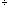what is 5'7" inches in cm? Hi, This is my height also, I am 5'7"tall. The conversion factor I remember is that there are 2.54 centimeters in 1 inch, so first I would change the feet to inches. 512 = 60 so 5'7" = 67". Let's make an estimate first and then do the calculations exactly. From above 1 inch is approximatelt 2.5 cm so 4 inches is approximately 42.5 = 10 cm. So every 4 inches of my height is approximately 10 cm. 674 is approximately 17 so I am approximately 1710 = 170 cm tall. Now let's do the calculations exactly. 5'7" is 67" and each inch is 2.54 cm, so 5'7" is 672.54 = 170.18 cm Wow! My approximations are not usually so close to the correct answer. Harley